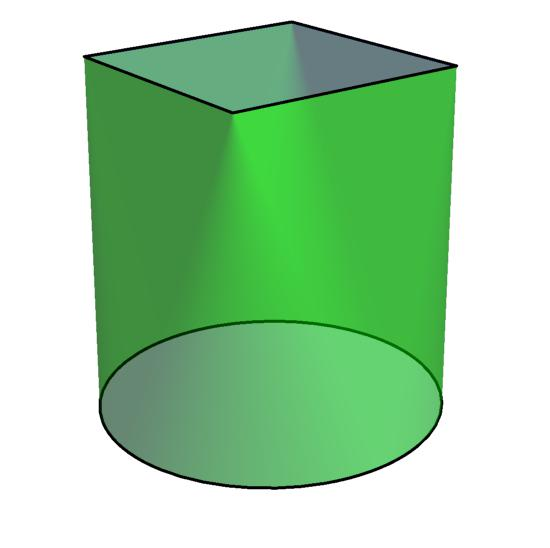# A Ruled Prism

Calculus Level 5Define a ruled prism to be a solid with 2 parallel faces and a ruled surface joining the 2 parallel faces continuously and without overlap.

Given that you know the perpendicular distance between the 2 parallel faces and the areas of $n$ cross-sections of the ruled prism, what is the minimum $n$ such that you can know the exact volume of the ruled prism?

(You can choose which cross-sections to find the area of.)

The diagram above is an example of a ruled prism with a square and a circle as the parallel faces and a ruled surface connecting them.

×

Problem Loading...

Note Loading...

Set Loading...